# Maths solutions for Applications of Trigonometry class 10 ssc

Tenth class mathematics chapter 12 exercises 12.1 and 12.2 solutions are given.

These solutions are very easy to understand. First study the chapter 12 well for many times.

Observe the example probems and solutions well. Try them in your own method. Next solve the exercise problems.

Observe the solutions and try them in your own method.

You can see the solutions for

2. Sets

10. Mensuration

11. Trigonometry

12. Applications of trigonometry

13. Probability

14. Statistics

# Applications of trigonometry

## Exercise 12.1 class 10 maths chapter 12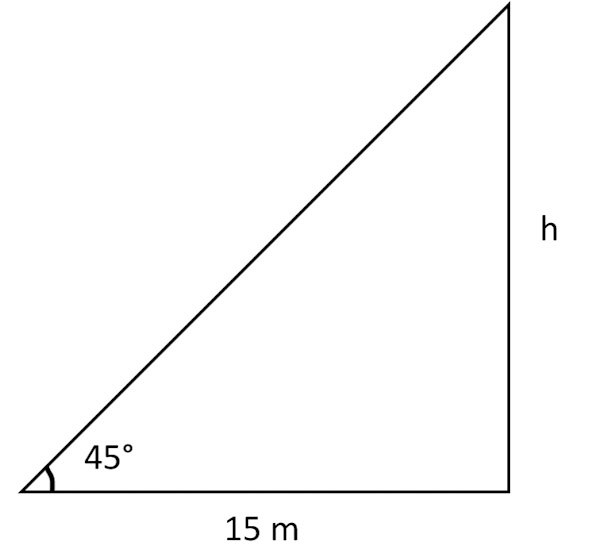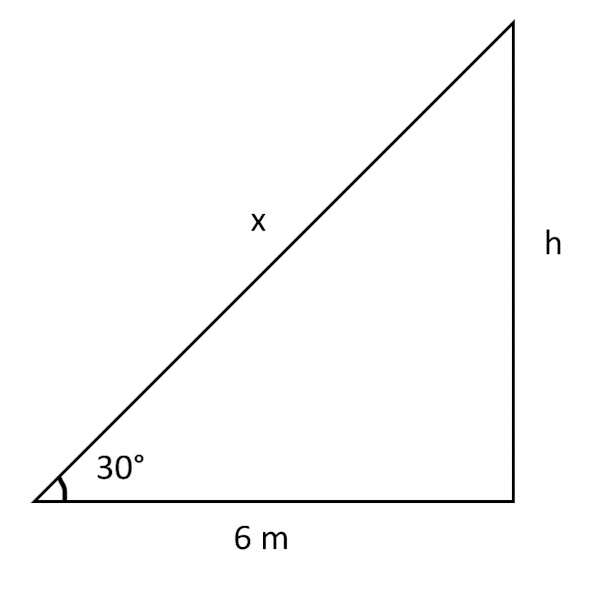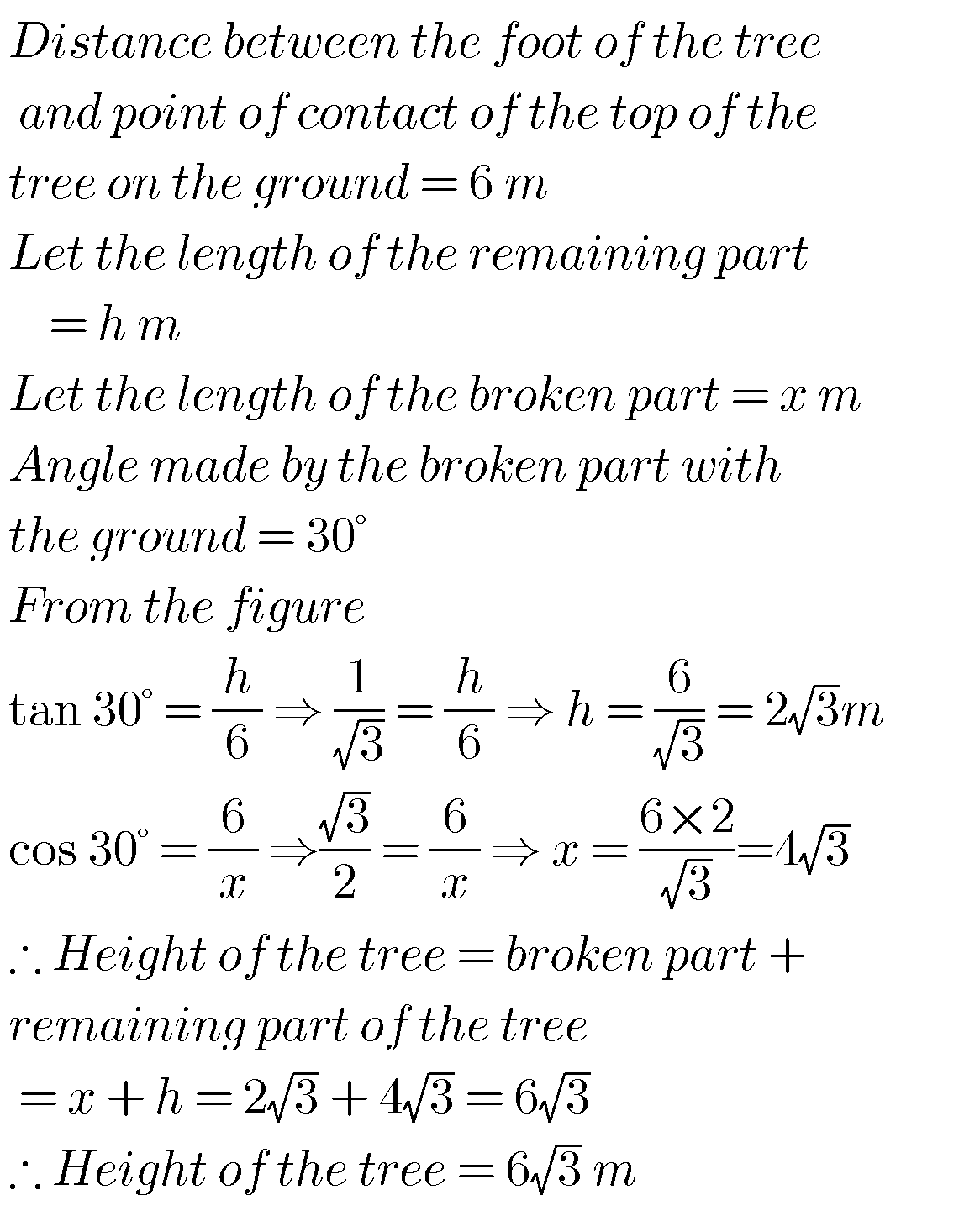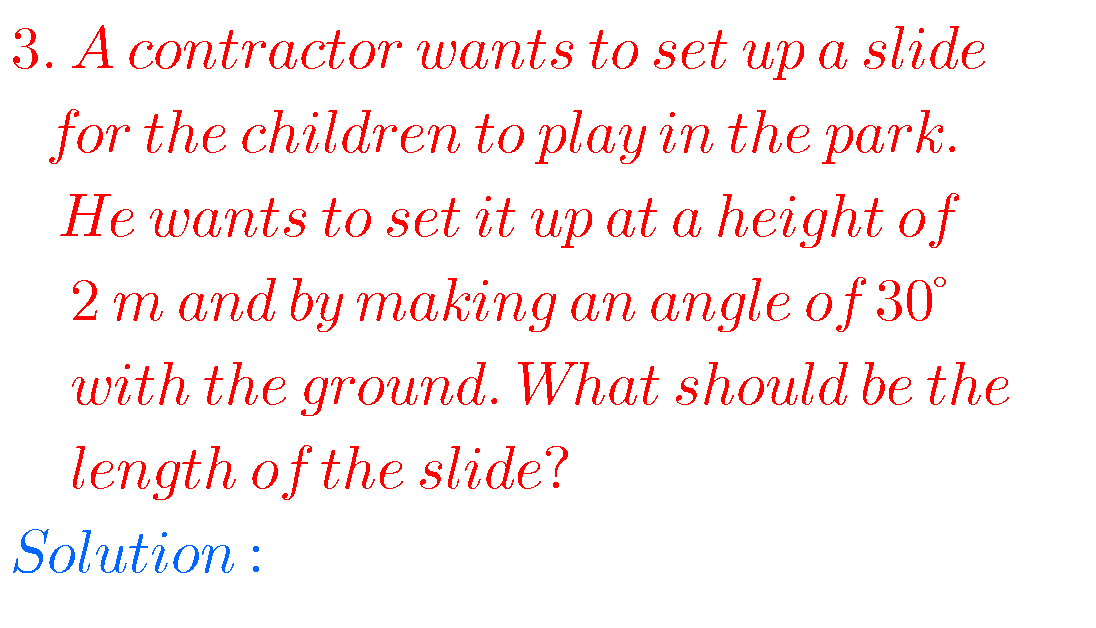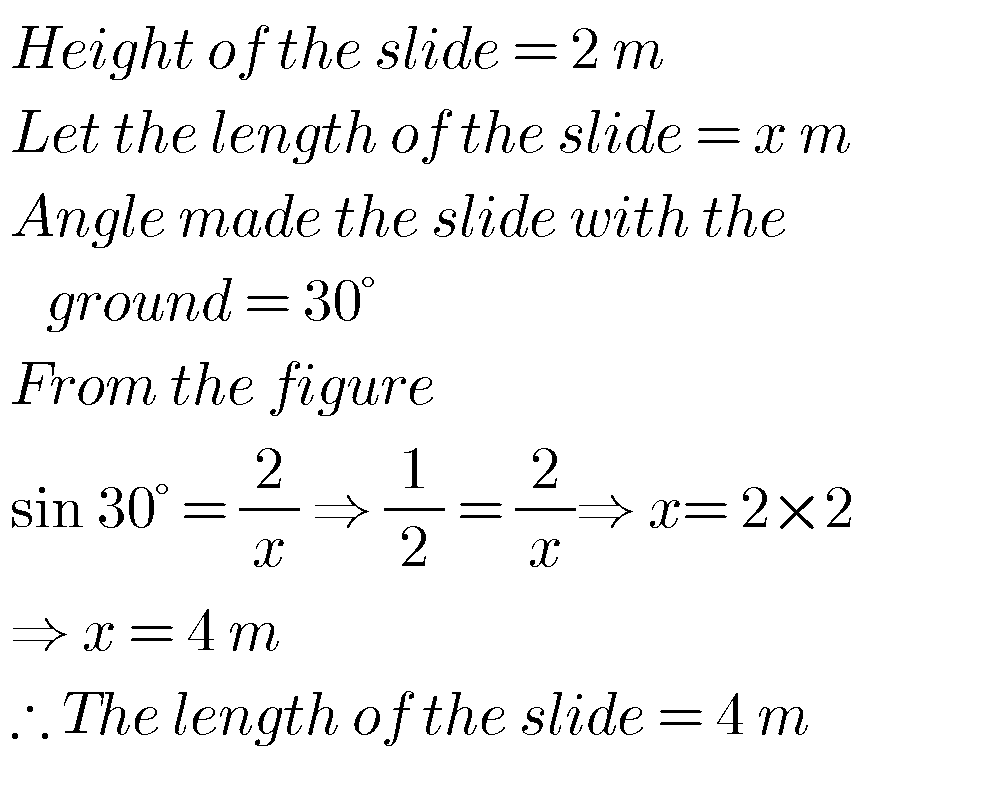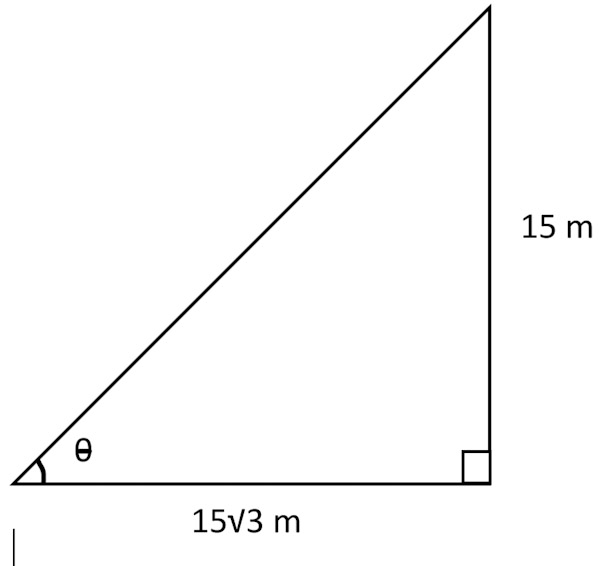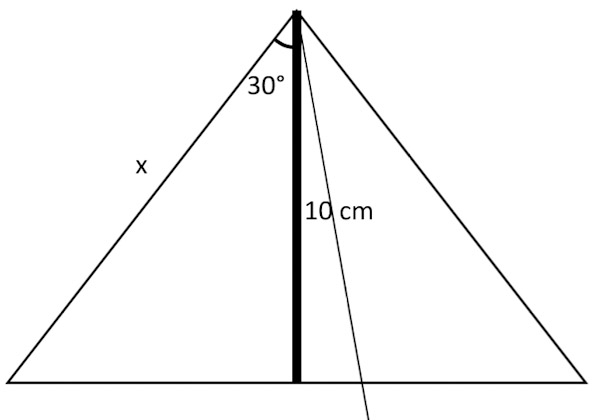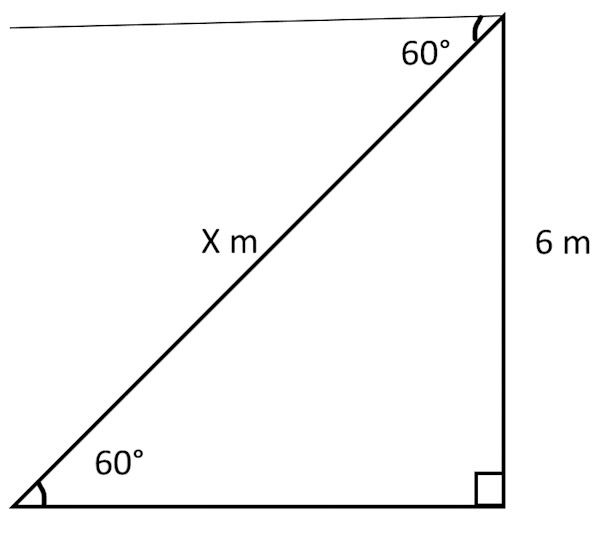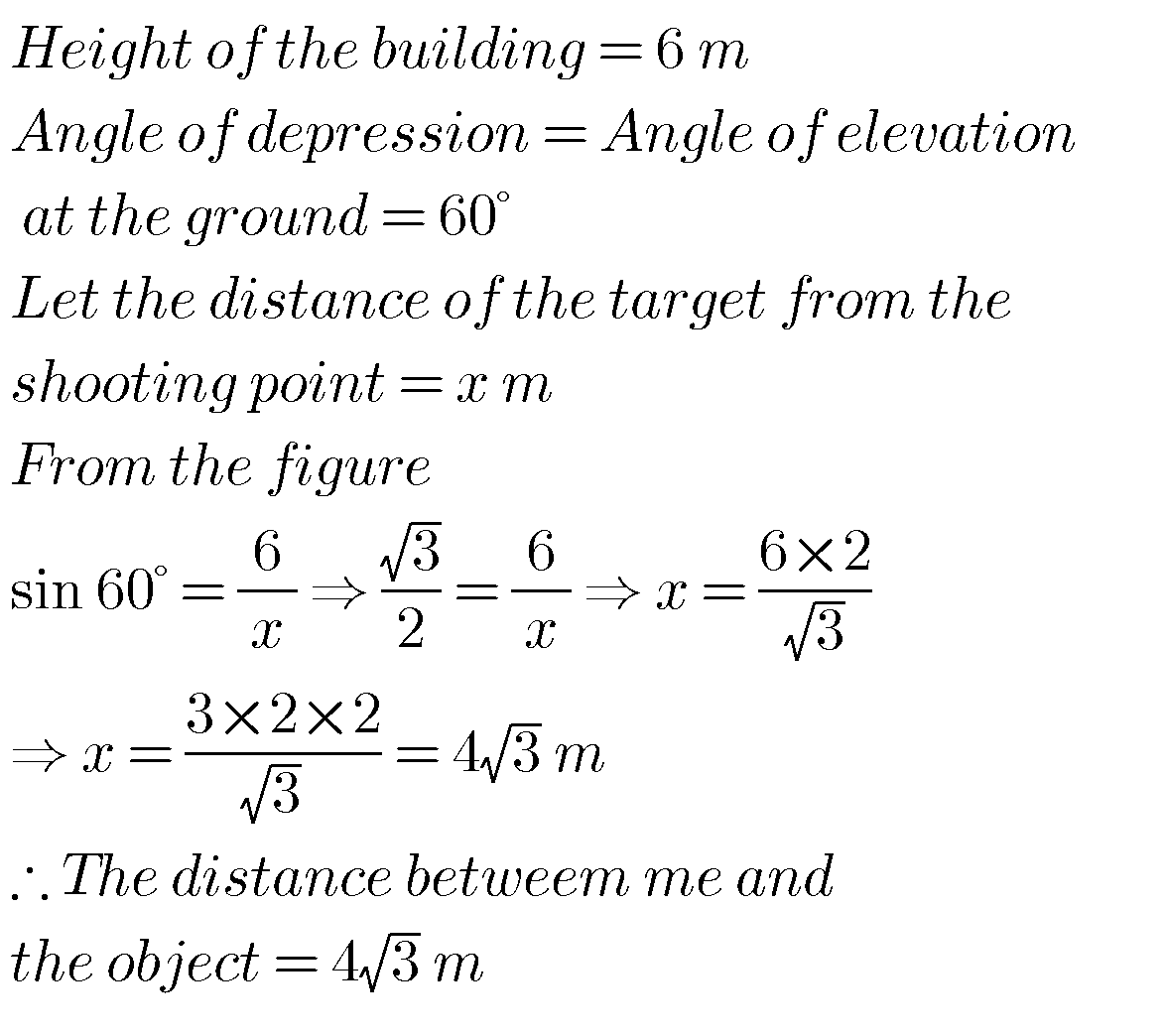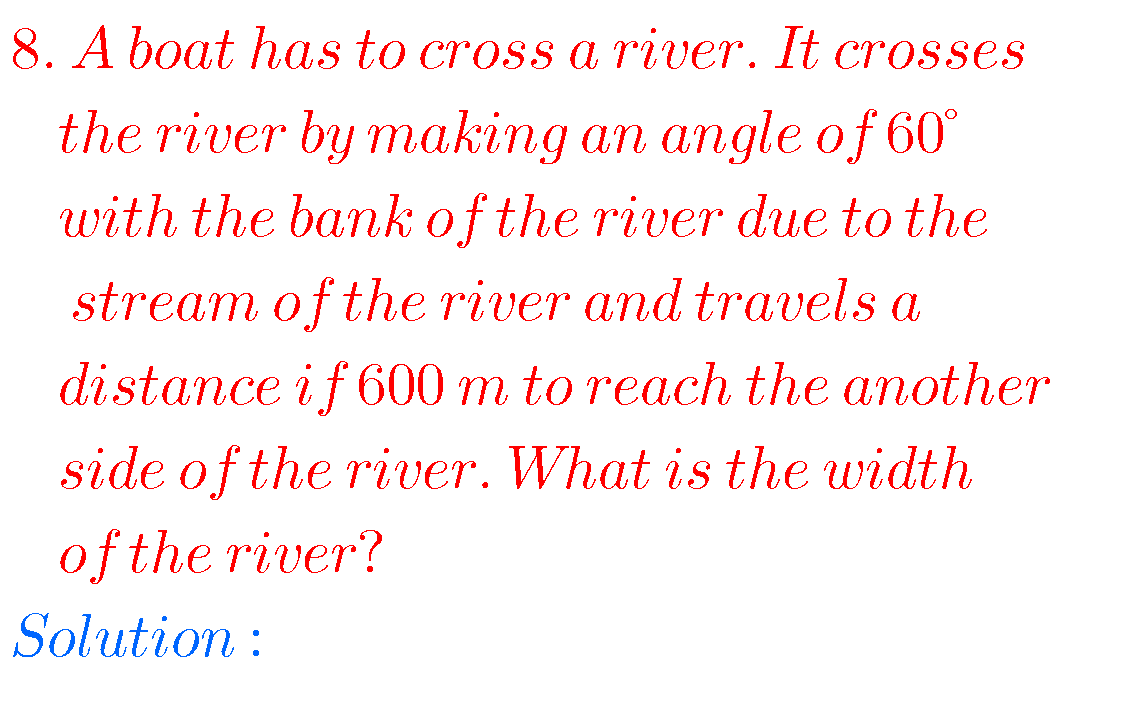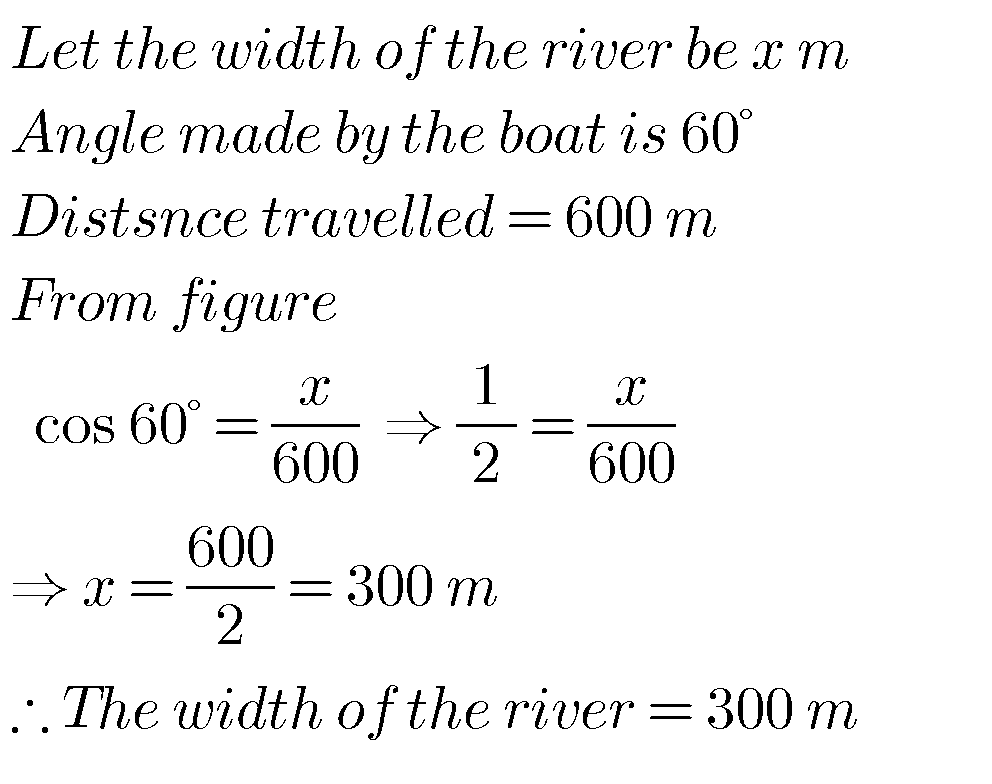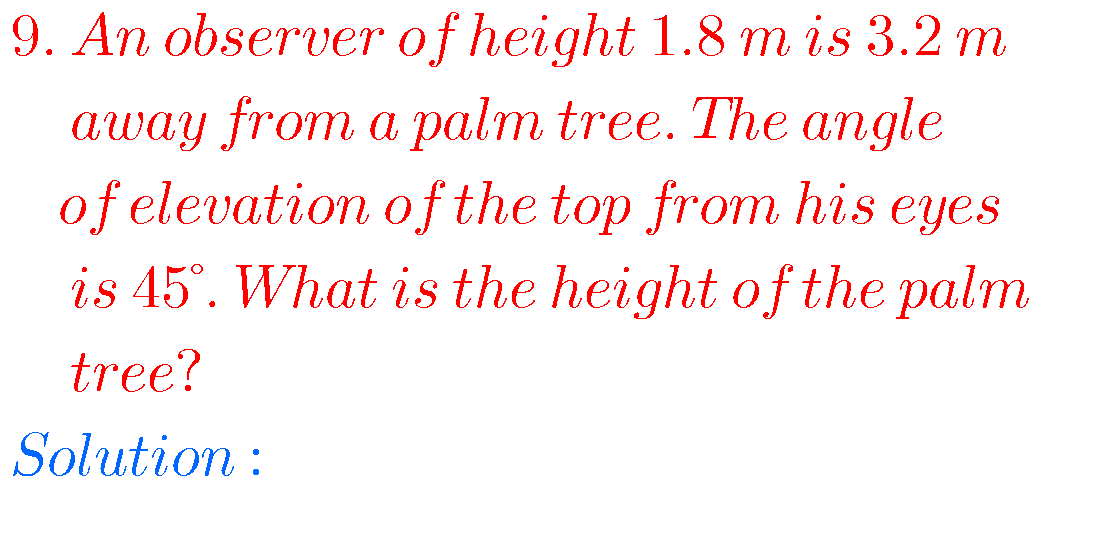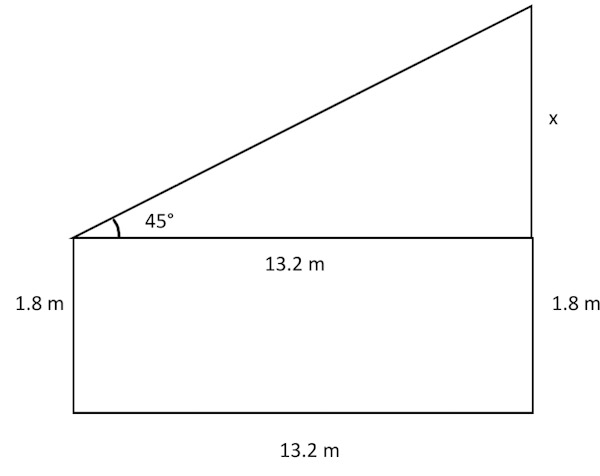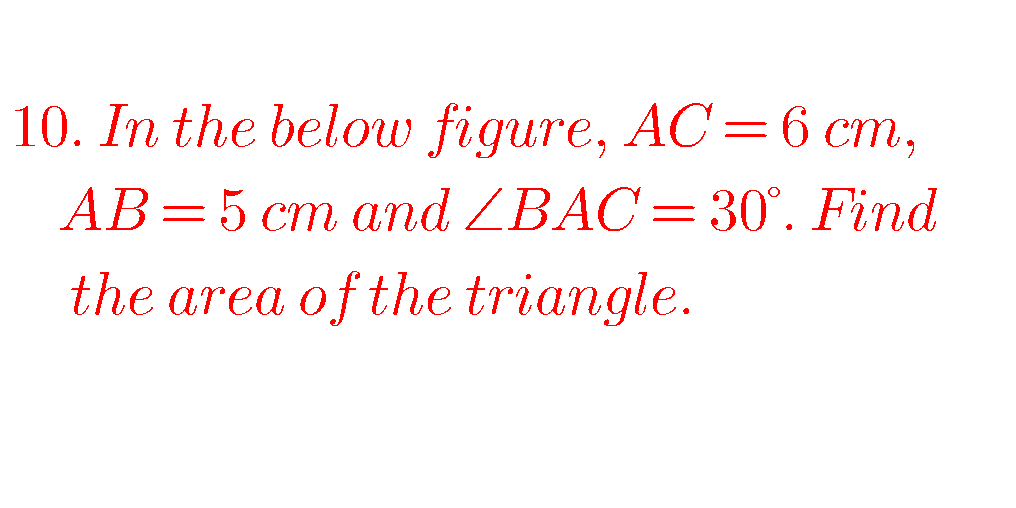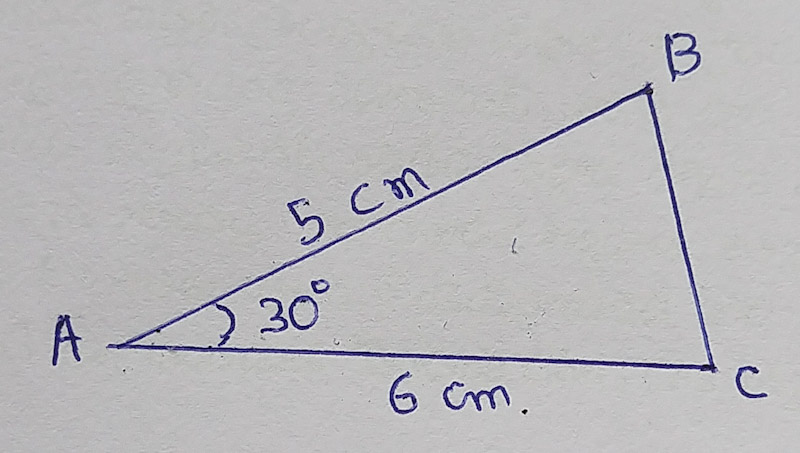## SSC Maths Applications of Trigonometry solutions

Exercise 12.2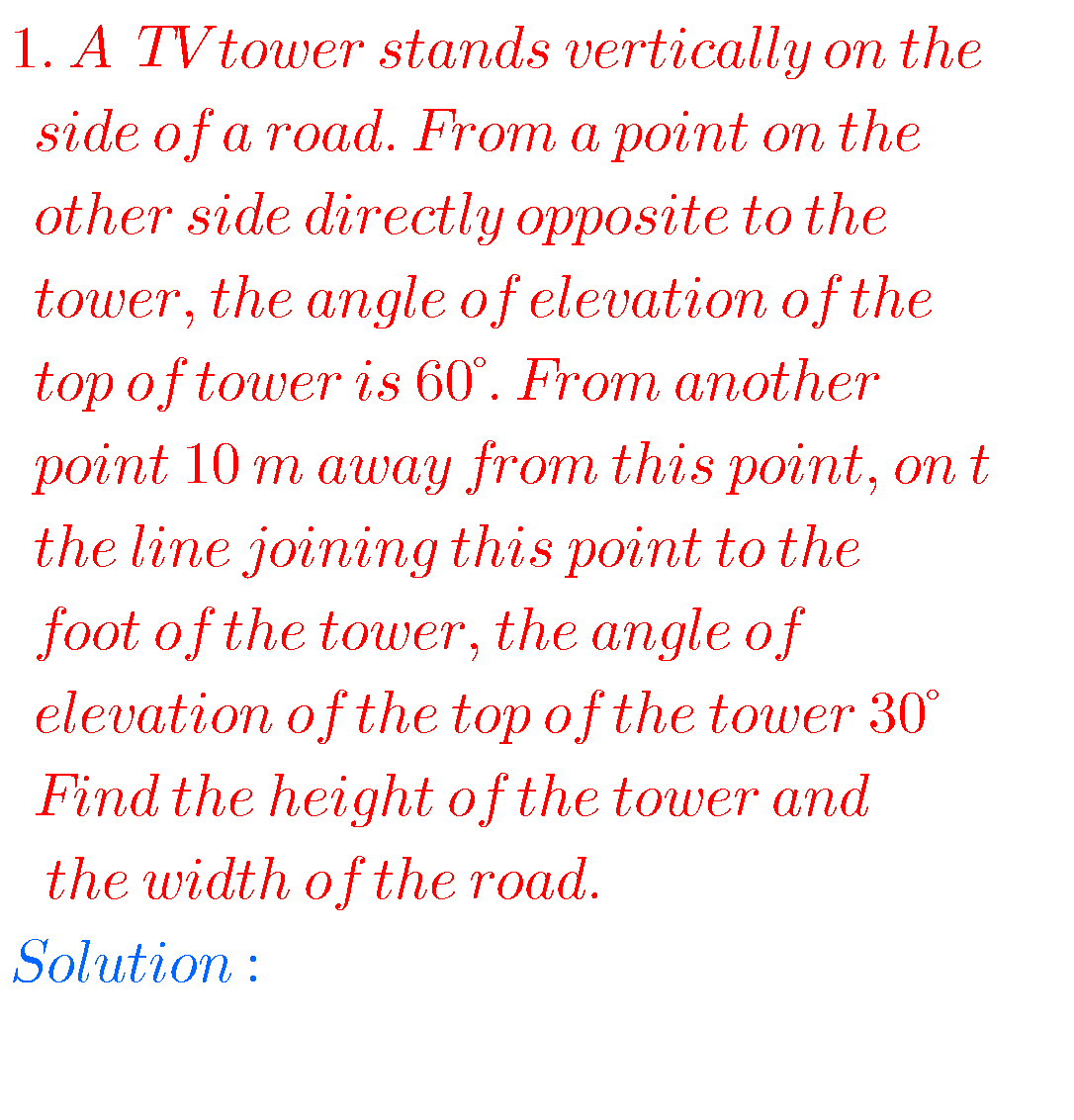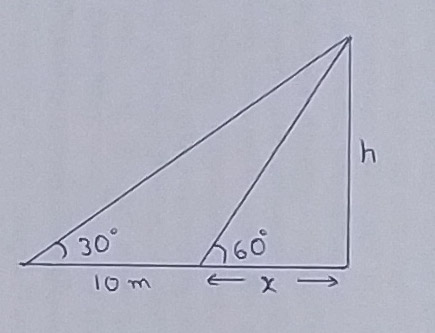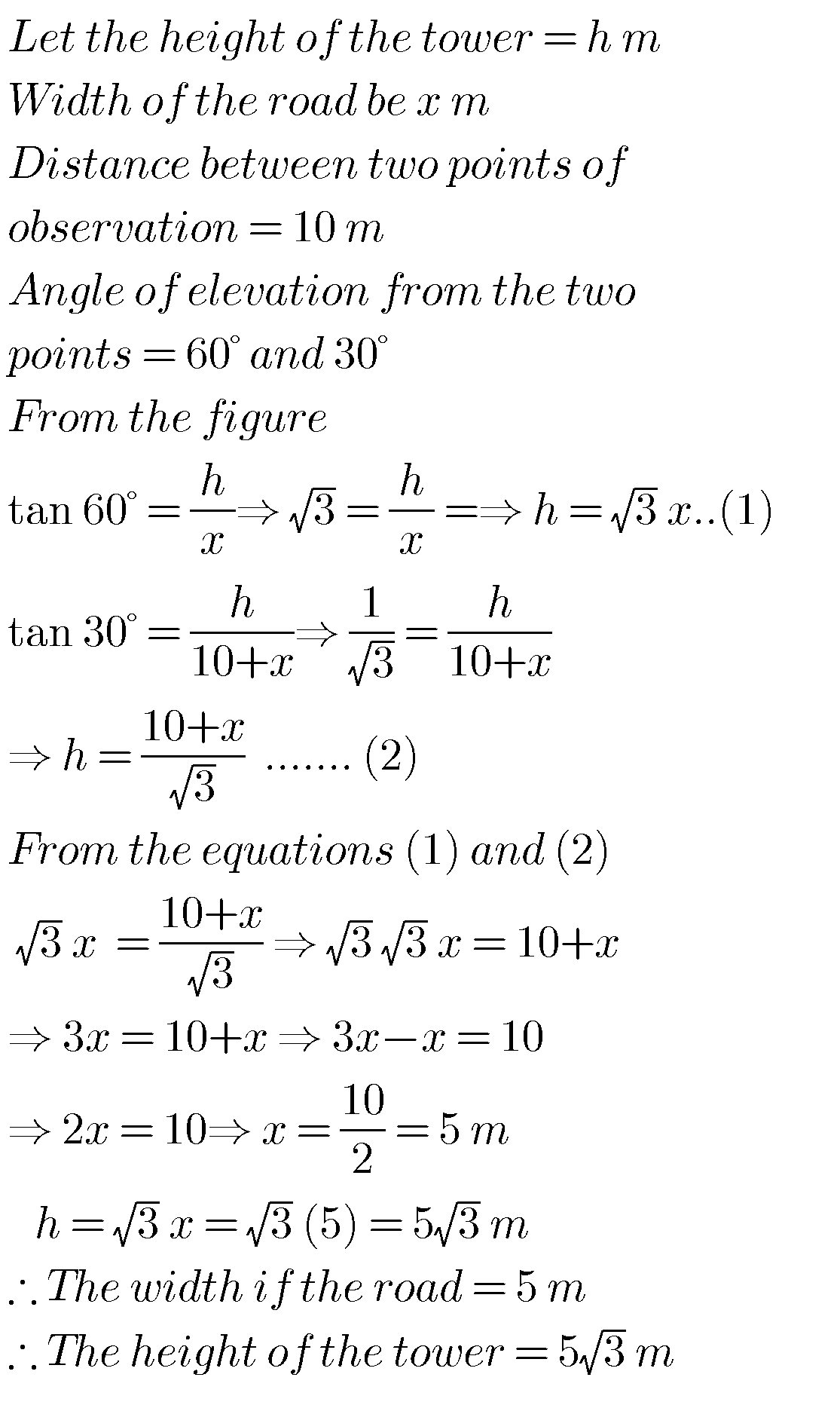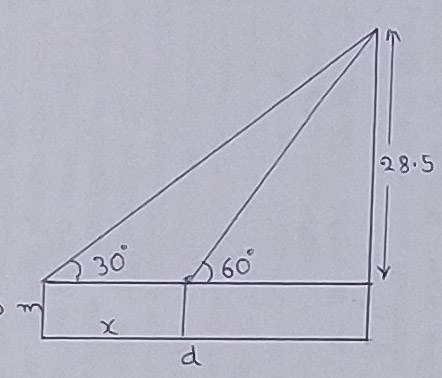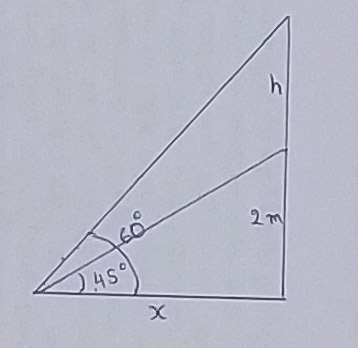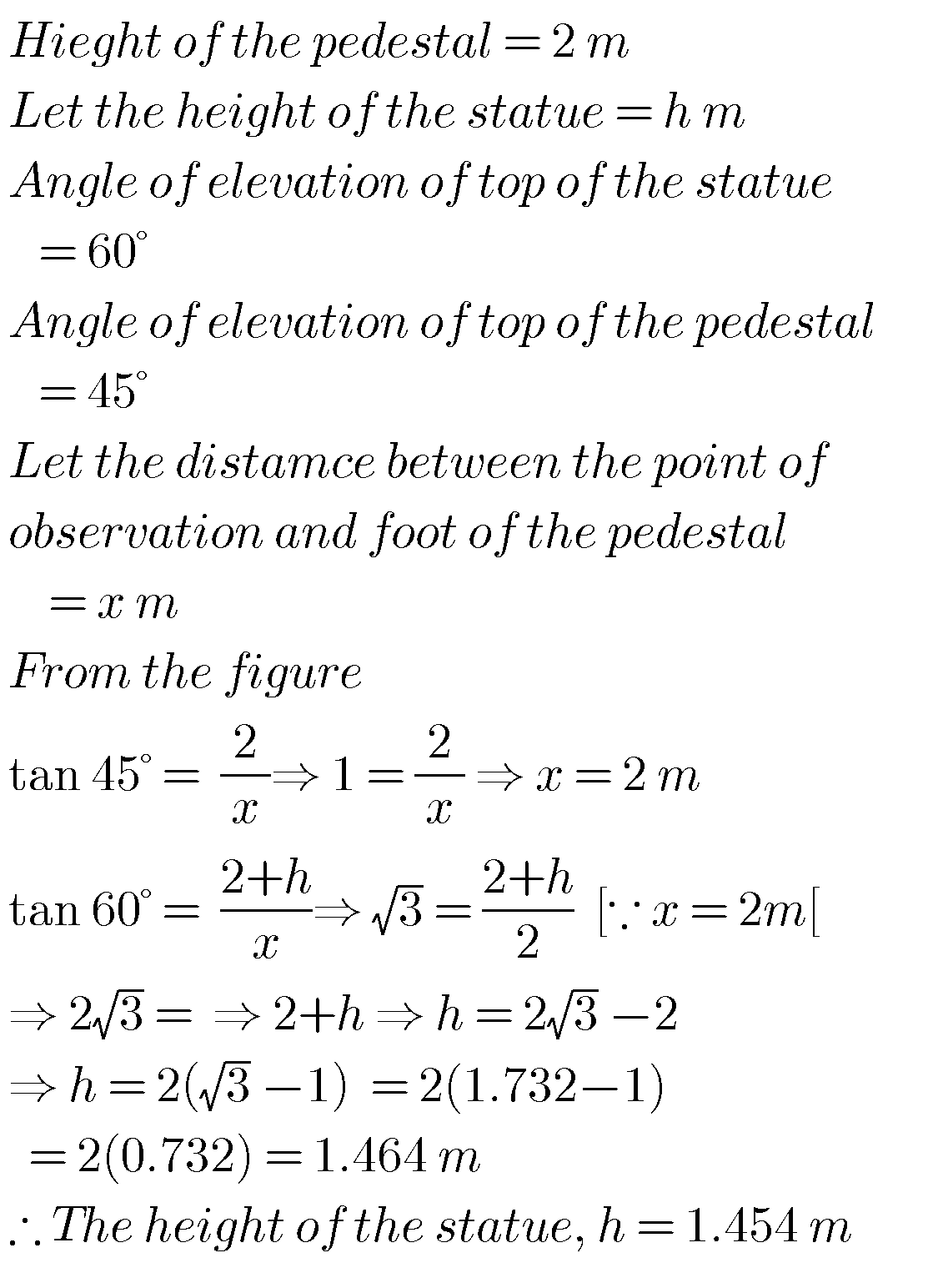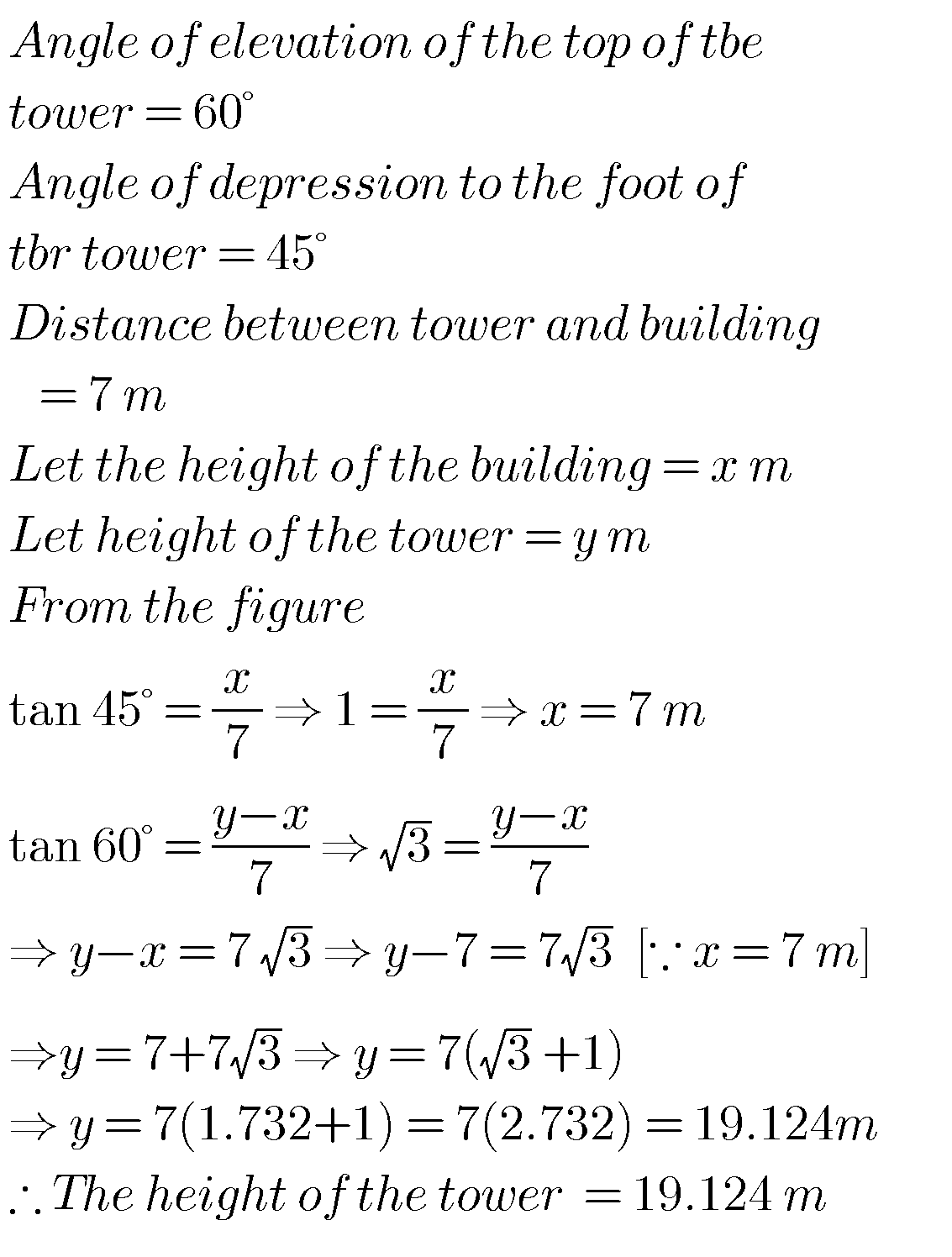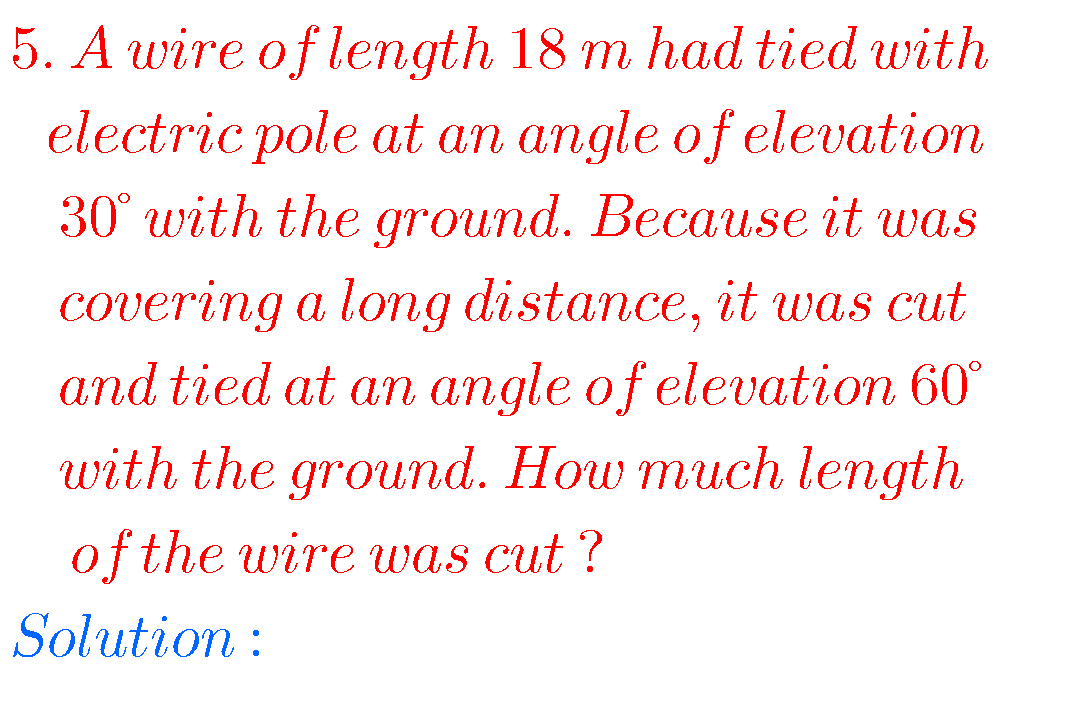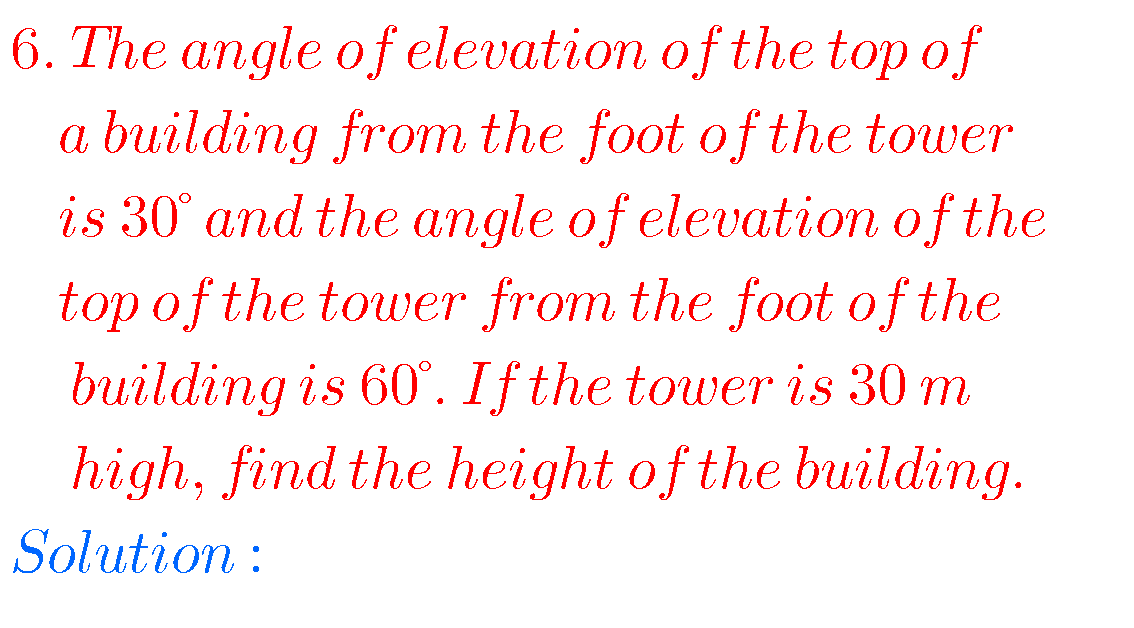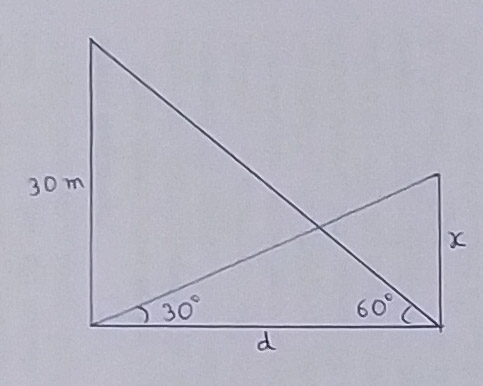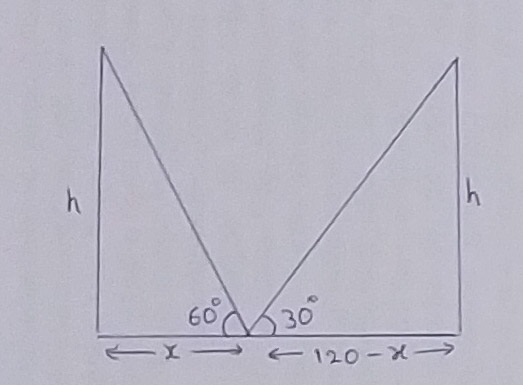###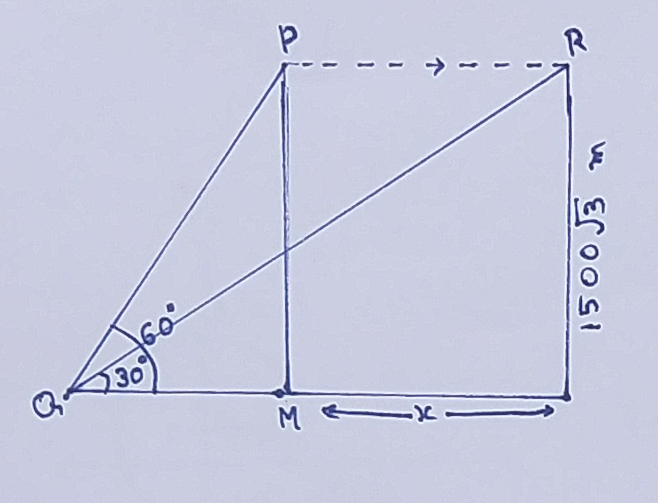###### Chapter 12 applications of trigonometry solutions class 10

Optional exercise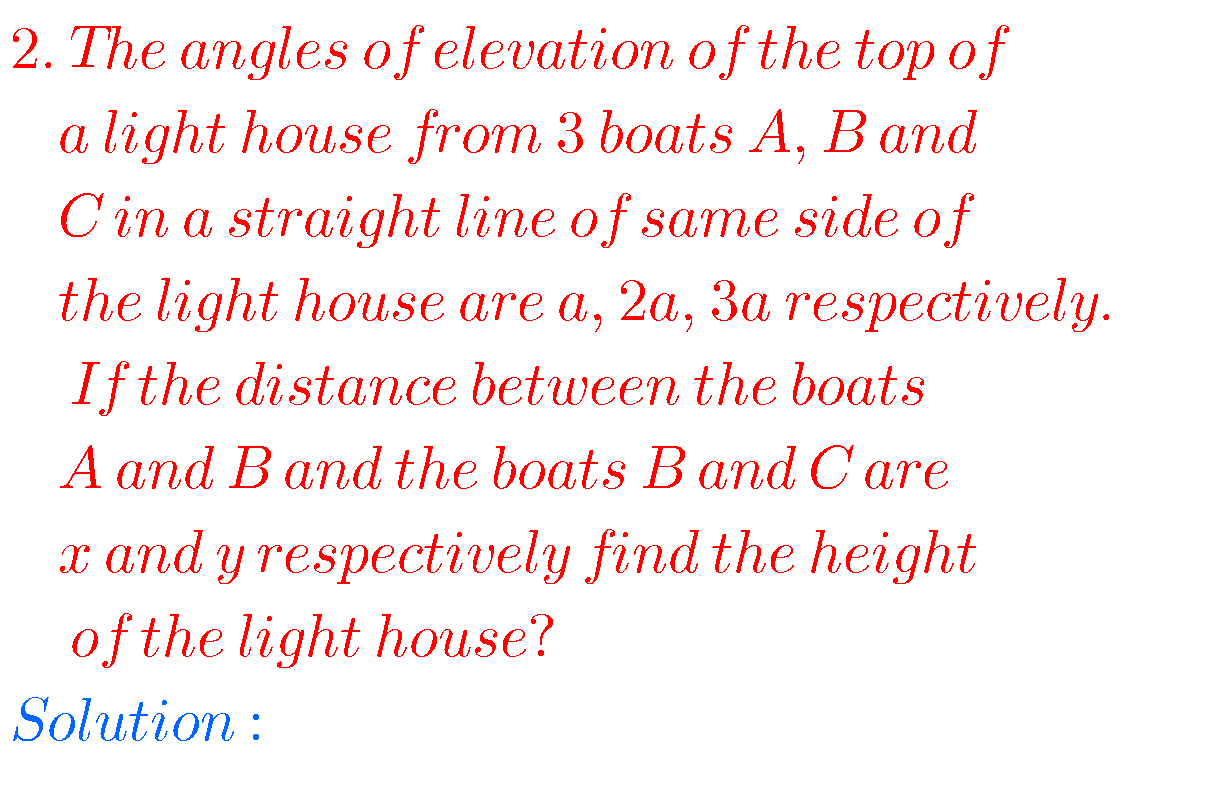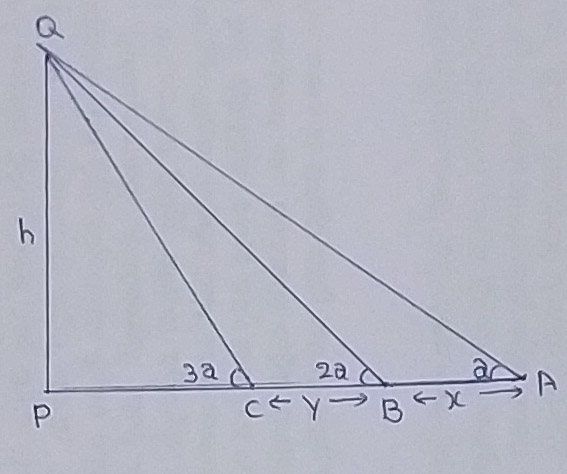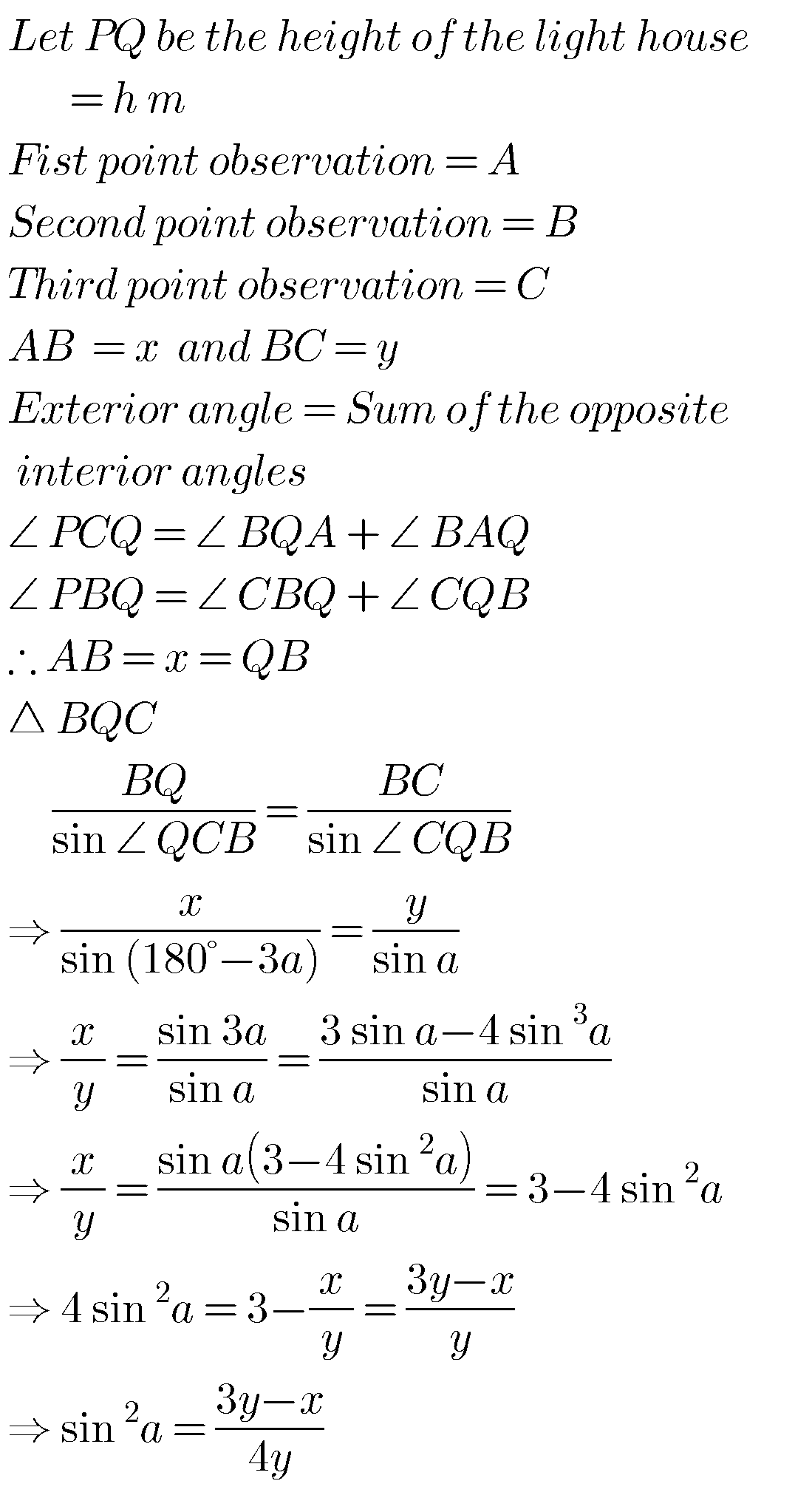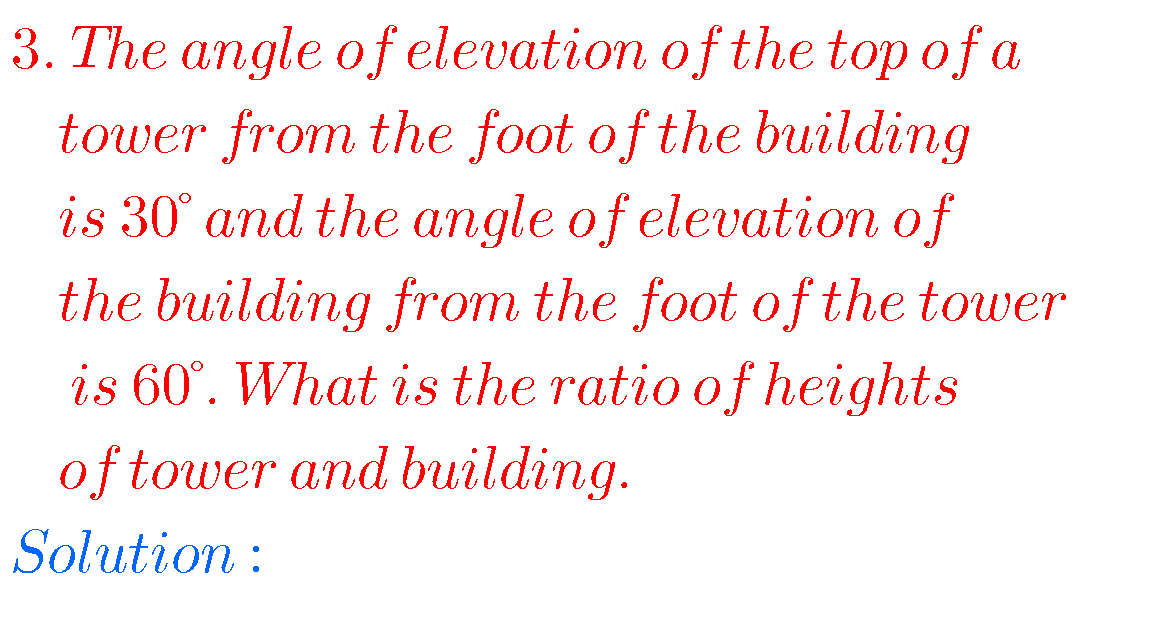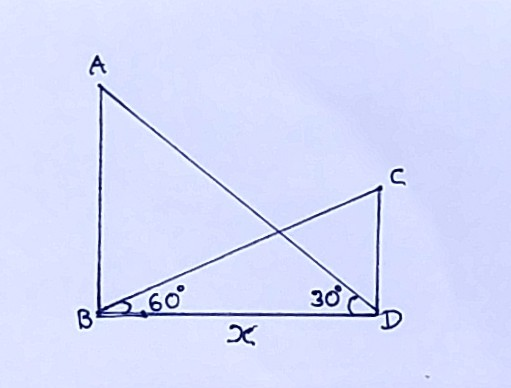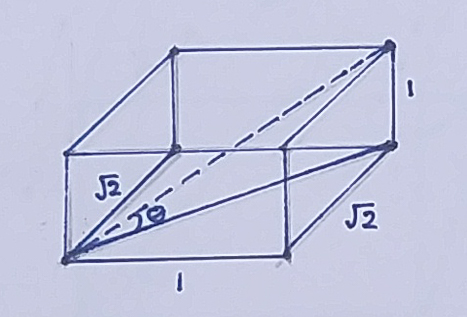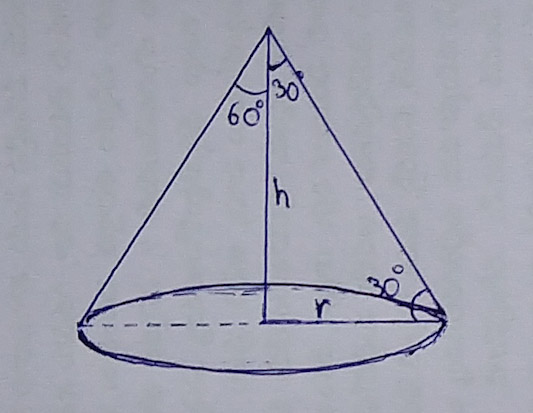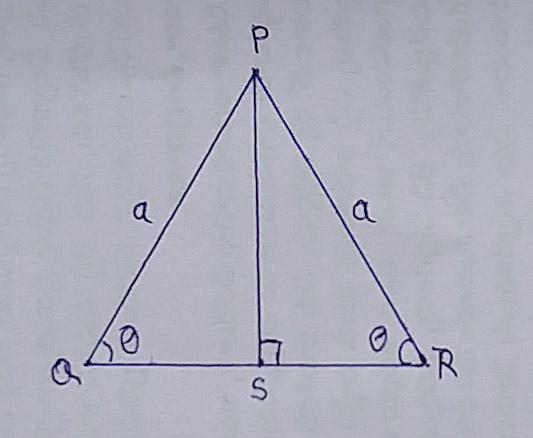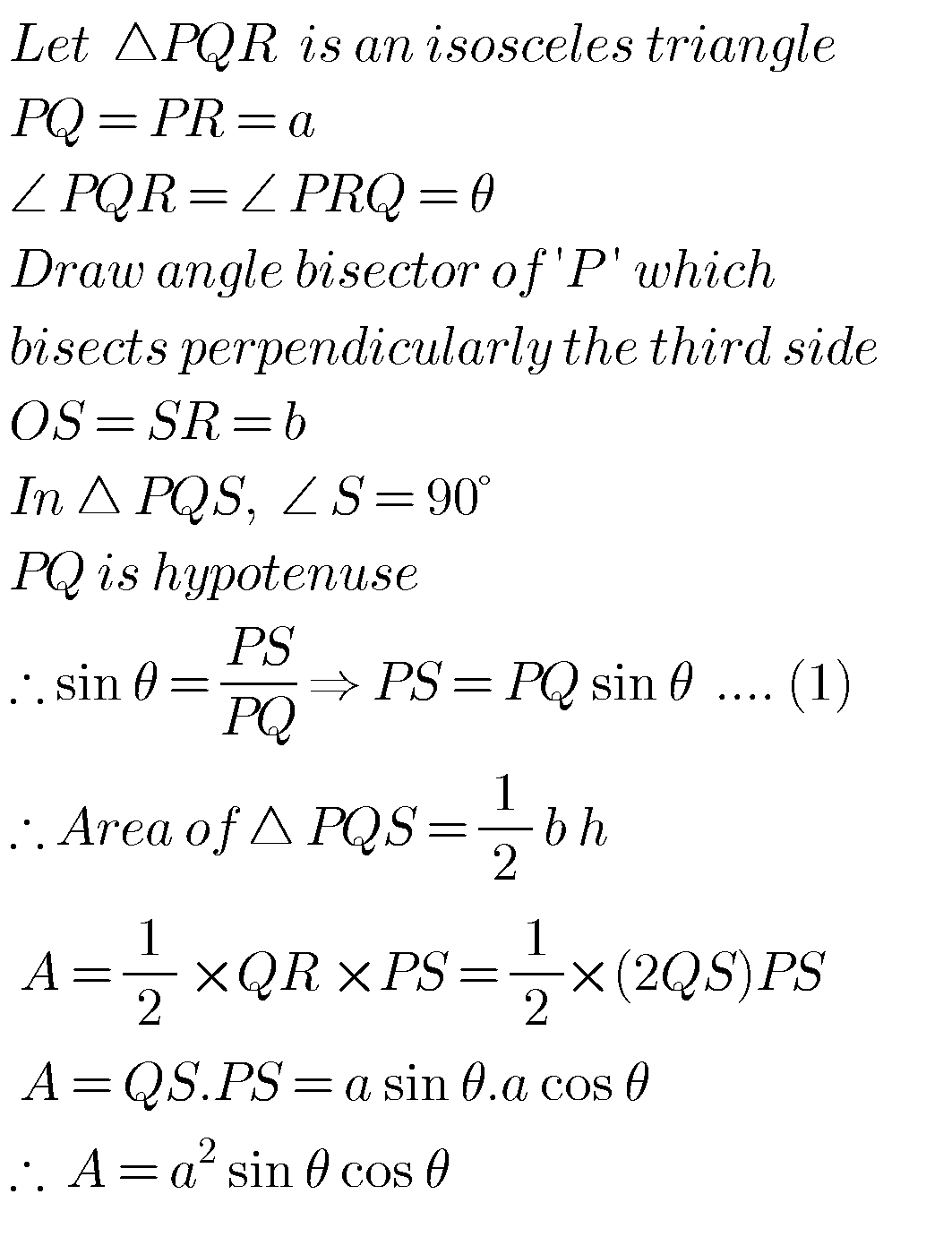Note : Observe the solutions and try them in your own methods.

Inter maths solutions for 1a matrices

Inter maths solutions for quadratic expressions

Ncert solutions for class 8 linear equations in two variables

Ncert solutions for class 7 rational numbers

Ncert solutions for class 7 simple equations

Ncert solutions for class 6 whole numbers

Ncert solutions for class 6 integers

You can see solutions for Inter Maths IIB

1. Circle

3. Parabola

4. Ellipse

You can also see solutions for Inter Maths IIA

For examination purpose you can see

Complex numbers

De Moivre’ s Theorem

Trigonometric Ratios upto Transformations

Exercise 6(a)

Exercise 6(b)

Exercise 6(c)